# 【C++】AVL树# 前言

`以下是本篇文章正文内容`

# 一、什么是AVL树？

AVL树也是一个二叉搜索树，只不过是在二叉搜索树的基础上，增加了一个条件：

# 二、AVL树的性质

• 1）它的左右子树都是AVL树
• 2）左右子树高度之差（平衡因子）的绝对值不超过1（-1/0/1）

# 三、二叉树节点的定义

``````template<class K, class V>
class AVLTreeNode
{
public:
AVLTreeNode(const pair<K, V> kv)
:_kv(kv)
,_left(nullptr)
,_right(nullptr)
,_parent(nullptr)
,_bf(0)
{}

pair<K, V> _kv;
AVLTreeNode<K, V>* _left;
AVLTreeNode<K, V>* _right;
AVLTreeNode<K, V>* _parent;
int _bf;
};
``````
• 1）需要有一个平衡因子存在，即`_bf`
• 2）_data值可以是一个键值对，也可以是其他类型，这里我选择了`pair`
• 3）在后面的其他操作中，会频繁用到一个节点的父亲，所以直接在节点中添加一个`_parent`成员。

# 四、AVL树的插入

1）先找到插入位置，因为这是一棵搜索树，如果该节点的值比根小，则往左走，如果比根大，往右边走。

2）找到待插入位置后，插入该节点，然后调整平衡因子。

## 旋转

### 1）右单旋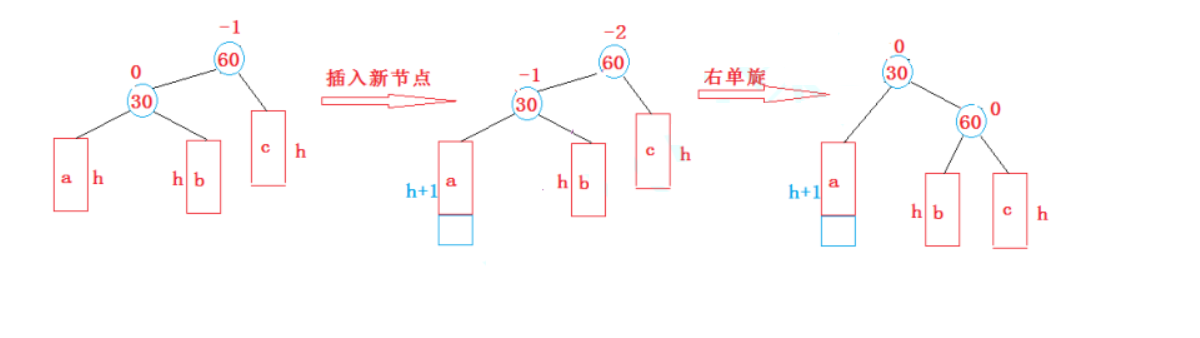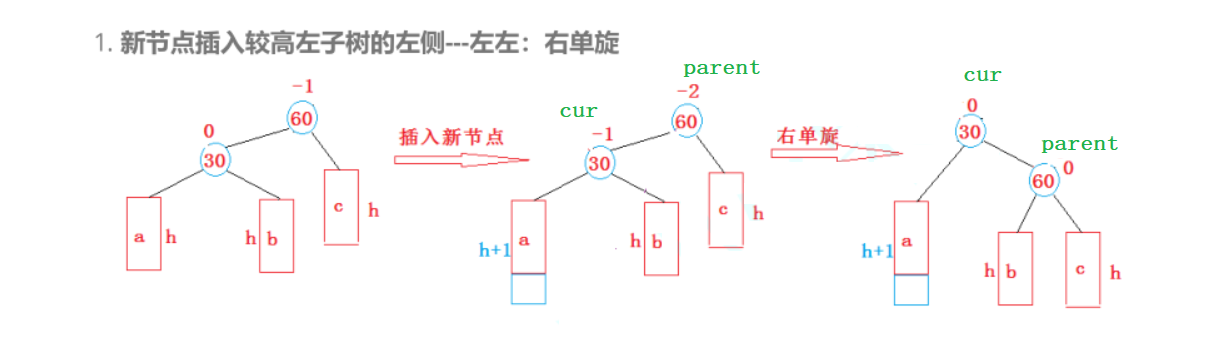• 1）cur的right给parent的left
• 2）parent变成了cur的right
调整后，cur变成了新的根，parent变成cur的right
此时需要注意一些细节：

### 2）左单旋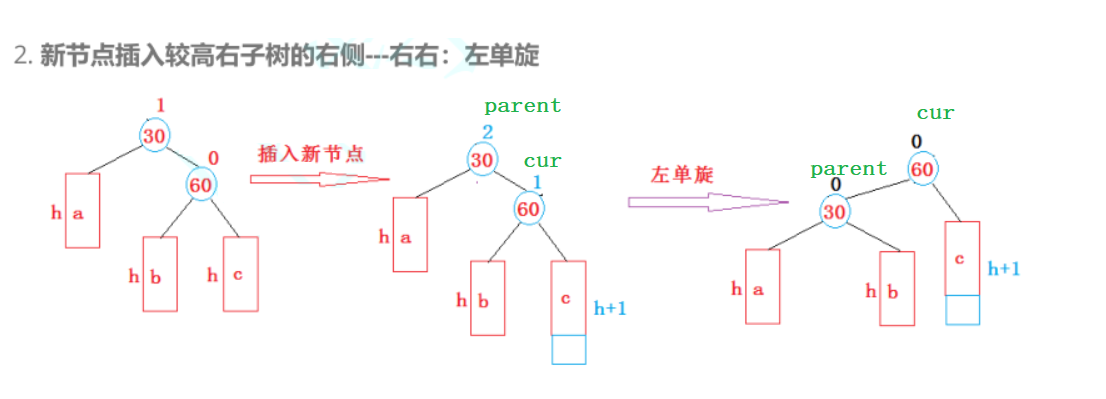• 1）cur的left给parent的right
• 2）parent变成了cur的left
调整后，cur变成了新的根，parent变成curr的left
此时需要注意一些细节：

### 3）左右双旋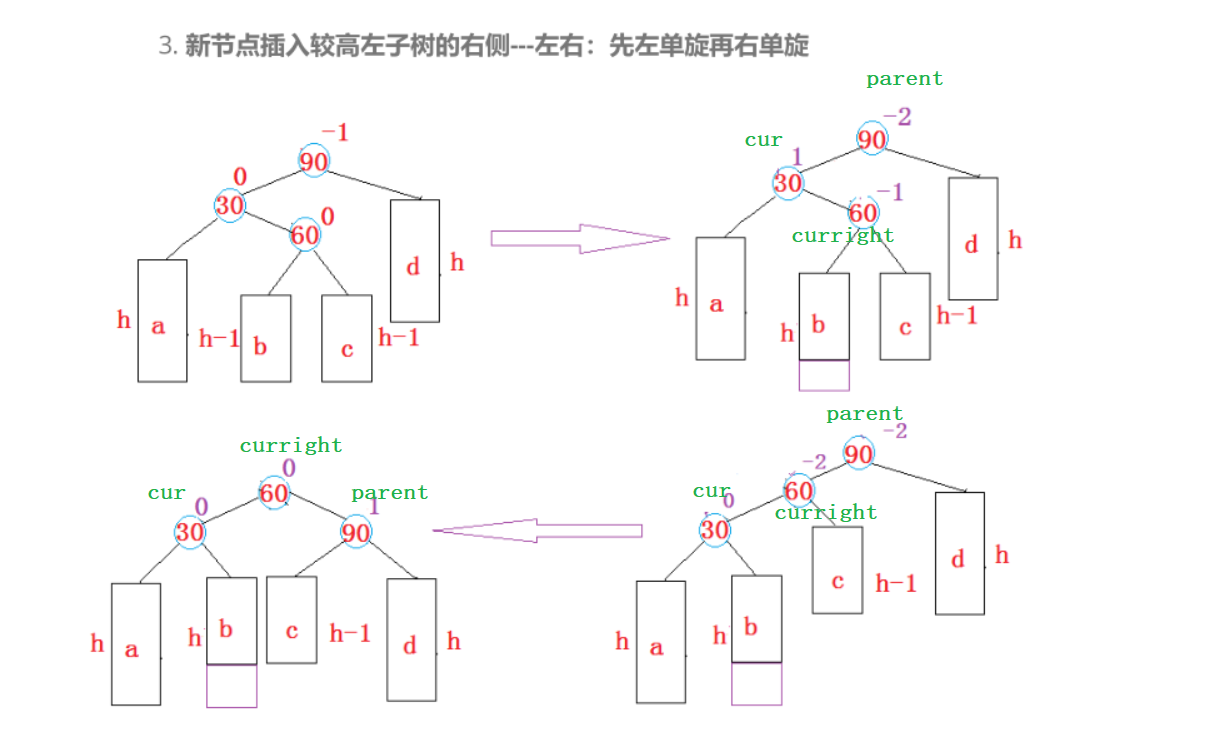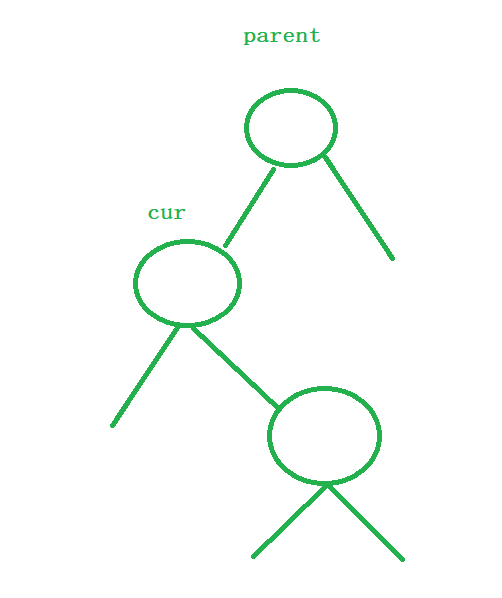• 1）让cur的右指向curright的左，然后cur成为curright的左。此时curright的父亲就变成了原来的cur的父亲，cur的父亲变成了curright

• 2）让parent的左指向curright的右，然后parent变成了curright的右，此时parent的父亲变成了curright。

• 1）如果旋转之前，curright的平衡因子是0，则说明，curright这个节点，一定是新插入的节点。

（因为如果不是新插入的节点，在插入该节点前，这棵树就不是AVL树了）。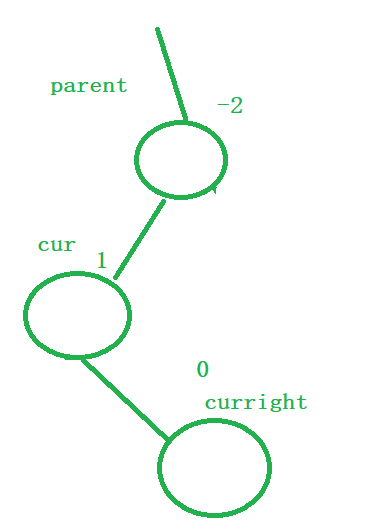• 2）如果旋转之前，curright这个节点的平衡因子是-1，该情况就是上图画出的情况，说明新插入节点在curright的左子树，在左右双旋后，cur和curright的平衡因子变成0，parent的平衡因子是1
• 3）如果旋转之前，curright这个节点的平衡因子是1，说明新插入节点在curright的右子树，在左右双旋后，parent和curright的平衡因子变成0，cur的平衡因子是-1

### 4）右左双旋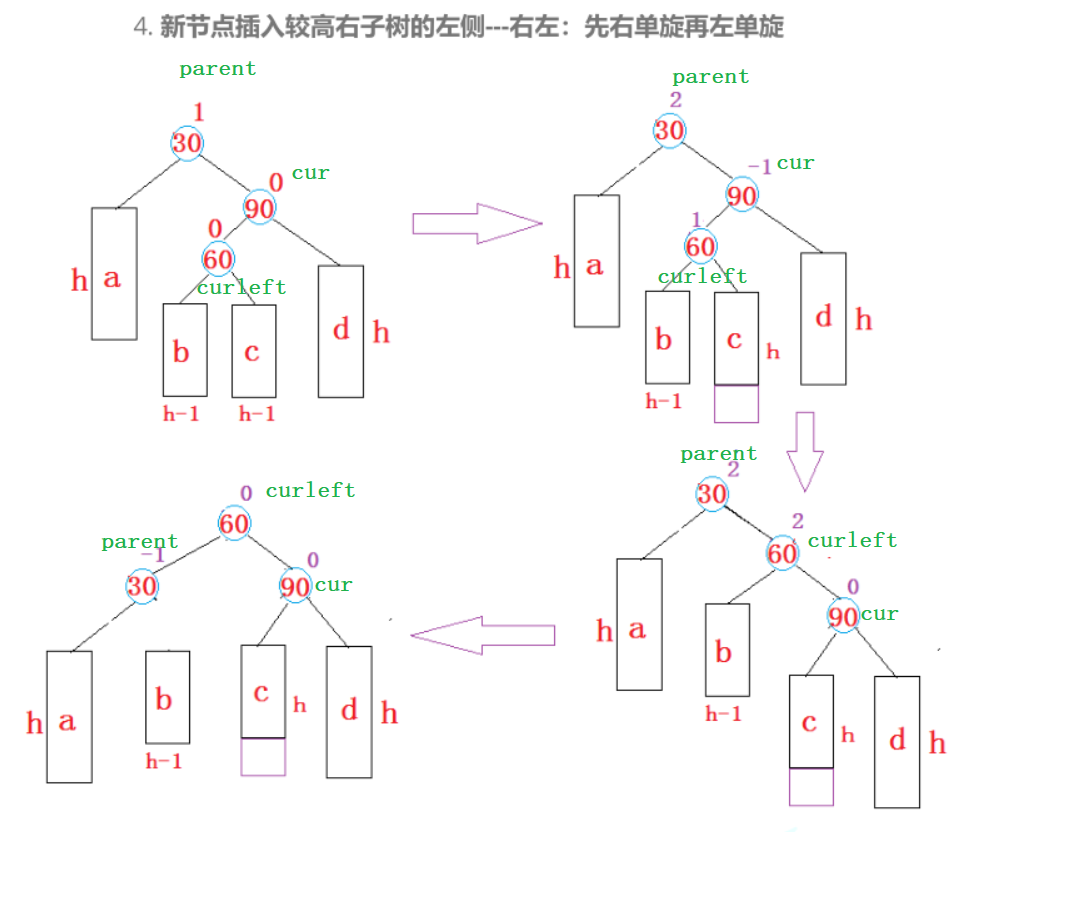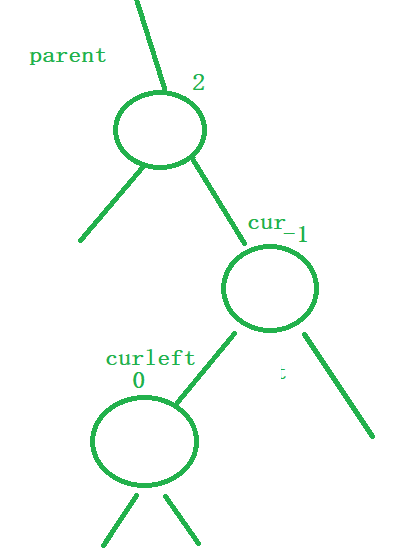• 1）让cur的左指向curleft的右，然后cur成为curleft的右。此时culeft的父亲就变成了原来的cur的父亲，cur的父亲变成了curleft

• 2）让parent的右指向curleft的左，然后parent变成了curleft的左，此时parent的父亲变成了curleft。

• 1）如果旋转之前，curleft的平衡因子是0，则说明，curleft这个节点，一定是新插入的节点。

（因为如果不是新插入的节点，在插入该节点前，这棵树就不是AVL树了）。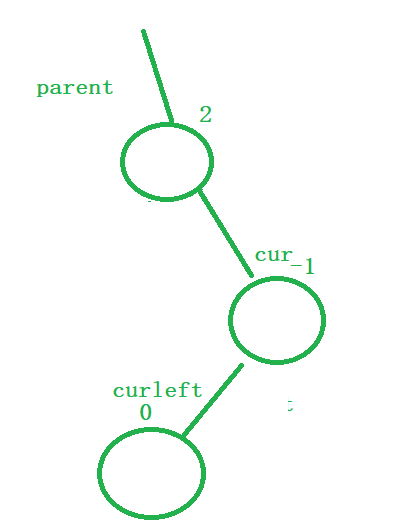• 2）如果旋转之前，curleft这个节点的平衡因子是1，该情况就是上图画出的情况，说明新插入节点在curleft的右子树，在右左双旋后，cur和curleft的平衡因子变成0，parent的平衡因子是-1
• 3）如果旋转之前，curleft这个节点的平衡因子是-1，说明新插入节点在curleft的左子树，在右左双旋后，parent和curleft的平衡因子变成0，cur的平衡因子是1

## AVL树插入完整代码

``````template<class K,class V>
class AVLTree
{
typedef AVLTreeNode<K, V> Node;
public:

AVLTree()
:_root(nullptr)
{}

bool Insert(const pair<K,V> kv)
{
if(_root == nullptr)
{
_root = new Node(kv);
return true;
}
Node* cur = _root;
Node* cur_parent = _root;
//1.找到待插入位置
while (cur)
{
if (cur->_kv.first < kv.first)
{
cur_parent = cur;
cur = cur->_right;
}
else if (cur->_kv.first > kv.first)
{
cur_parent = cur;
cur = cur->_left;
}
else
return false;
}

//2.先判断待插入节点是在parent的左边还是右边
cur = new Node(kv);
if (cur_parent->_kv.first > kv.first)
{
cur_parent->_left = cur;
}
else
{
cur_parent->_right = cur;
}
cur->_parent = cur_parent;

//下面为调整二叉树的平衡

//1.更新平衡因子
//
while (cur_parent)
{
//左子树--
if (cur_parent->_left == cur)
{
cur_parent->_bf--;
}
//右子树++
else
{
cur_parent->_bf++;
}

//平衡因子=0，不再影响祖先
if (cur_parent->_bf == 0)
{
break;
}

//不平衡了，影响祖先，要向上也调整
else if (cur_parent->_bf == 1 || cur_parent->_bf == -1)
{
cur = cur_parent;
cur_parent = cur_parent->_parent;
}

//此时该树出问题了，需要旋转进行平衡

else if (cur_parent->_bf == 2 || cur_parent->_bf == -2)
{
//右边高，向左旋转
if (cur_parent->_bf == 2 && cur->_bf == 1)
{
RotateL(cur_parent);
}

//左边高，向右旋转
else if (cur_parent->_bf == -2 && cur->_bf == -1)
{
RotateR(cur_parent);
}

//折线型，右边高，右左双旋
else if (cur_parent->_bf == 2 && cur->_bf == -1)
{
RotateRL(cur_parent);
}

//折线形，左边高，左右双旋
else if (cur_parent->_bf == -2 && cur->_bf == 1)
{
RotateLR(cur_parent);
}

//旋转完成后一定平衡了，则break
break;

}

else
{
assert(false);
}

}
cout << kv.first << endl;
return true;
}

//左单旋
void RotateL(Node* parent)
{
Node* cur = parent->_right;
Node* curleft = cur->_left;
Node* ppNode = parent->_parent;

parent->_right = curleft;
if (curleft)
curleft->_parent = parent;

cur->_left = parent;
parent->_parent = cur;

if (!ppNode)
{
_root = cur;
cur->_parent = nullptr;
}
else
{
if (ppNode->_right == parent)
{
ppNode->_right = cur;
}
else
{
ppNode->_left = cur;
}

cur->_parent = ppNode;
}

cur->_bf = parent->_bf = 0;

}

//右单旋
void RotateR(Node* parent)
{
Node* cur = parent->_left;
Node* curright = cur->_right;
Node* ppNode = parent->_parent;

parent->_left = curright;
//如果cur的右子树是空
if(curright)
curright->_parent = parent;

cur->_right = parent;
parent->_parent = cur;

if (!ppNode)
{
_root = cur;
cur->_parent = nullptr;
}
else
{
cur->_parent = ppNode;
//要知道根的左边是cur还是右边是cur
if (ppNode->_left == parent)
{
ppNode->_left = cur;
}
else
{
ppNode->_right = cur;
}
}

cur->_bf = parent->_bf = 0;
}

void RotateLR(Node* parent)
{
Node* cur = parent->_left;
Node* curright = cur->_right;
int bf = curright->_bf;

RotateL(parent->_left);
RotateR(parent);

//该节点为新插入的节点
if (bf == 0)
{
curright->_bf = 0;
cur->_bf = 0;
parent->_bf = 0;
}

//新插入节点在curright的右边，右边高了
//     p
//   c
//     cr

else if (bf == 1)
{
curright->_bf = 0;
cur->_bf = -1;
parent->_bf = 0;
}

//新插入节点在curright的左边，左边高了
else if (bf == -1)
{
curright->_bf = 0;
cur->_bf = 0;
parent->_bf = 1;
}

}

void RotateRL(Node* parent)
{
Node* cur = parent->_right;
Node* curleft = cur->_left;
int bf = curleft->_bf;

RotateR(parent->_right);
RotateL(parent);

//该节点为新插入的节点
if (bf == 0)
{
curleft->_bf = 0;
cur->_bf = 0;
parent->_bf = 0;
}
//新插入节点在curleft的右边，右边高了
//     p
//		  c
//     cl
else if (bf == 1)
{
curleft->_bf = 0;
cur->_bf = 0;
parent->_bf = -1;
}
//新插入节点在curleft的左边，左边高了
else if (bf == -1)
{
curleft->_bf = 0;
cur->_bf = 1;
parent->_bf = 0;
}
}

private:
Node* _root = nullptr;
};
``````

## 验证一棵树为AVL树

• 1）验证这棵树的中序遍历是有序的。
• 2）验证每一棵树的左右子树高度差不大于1
``````int Height(Node* root)
{
if (root == nullptr)
return 0;

int left = Height(root->_left);
int right = Height(root->_right);
return left > right ? left + 1 : right + 1;
}

bool IsBalanceTree()
{
cout << "IsBalanceTree():";
return _IsBalanceTree(_root);
}

//通过高度判断是否为AVL树
//1.通过高度计算出真实的平衡因子，再与AVL树本身的平衡因子进行比较

bool _IsBalanceTree(Node* root)
{
if (root == nullptr)
return true;

int lefth = Height(root->_left);
int righth = Height(root->_right);
int bf = righth - lefth;

if (bf != root->_bf || bf > 1 || bf < -1)
{
return false;
}

return _IsBalanceTree(root->_left) && _IsBalanceTree(root->_right);
}
``````

# 总结

### 网站公告